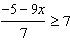# Algebra 2 Warmups 1.7

Solve the following inequalities.  <= means less than or equal to

one    11x – 9 > 24

two   12x – 7 <= 4x + 25

threefour   9 < -3(-5x + 7)

five   13x – 2(3x+5) <= 6 + 4(x-3)

<= means less than or equal to in computer coding. You can write it with a line under the less than symbol.

Answers (one  x > 3, two  x<=4, three  x <= -6, four  x > 2, five  x <= 4/3 or 1.3 repeating)

(source)